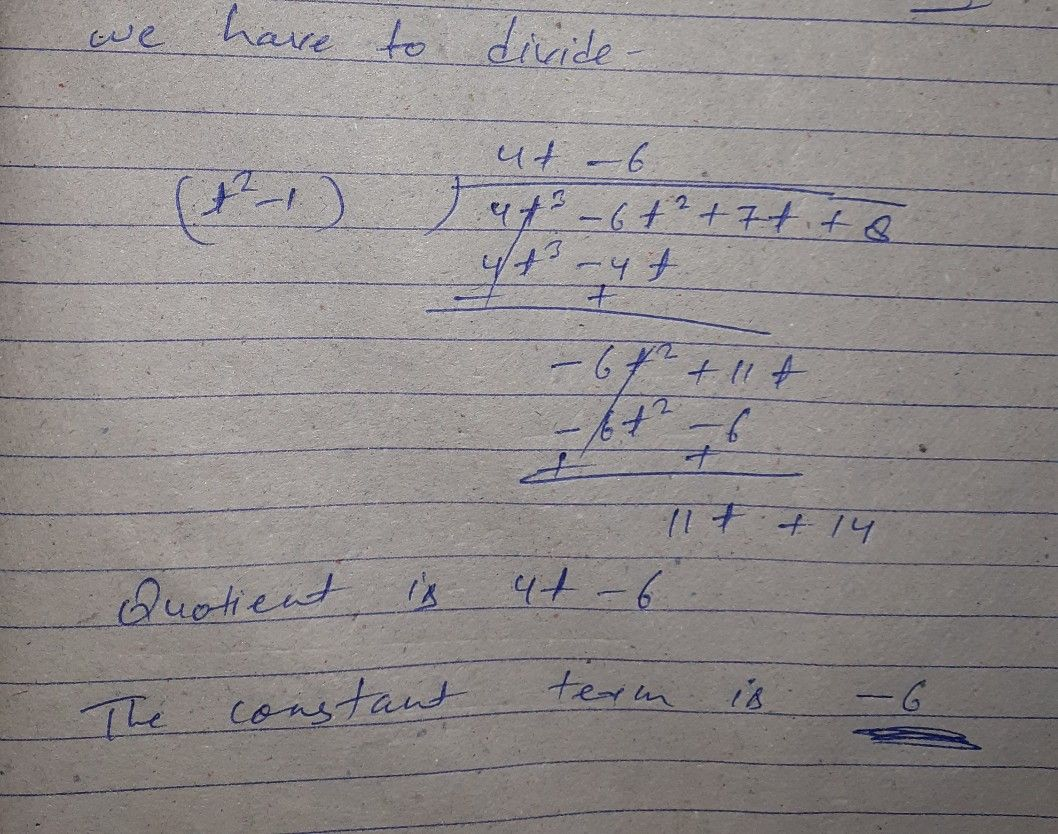Symbol
Problem$026$ of total $50$ questions Not A $5$ $\left(4t^{3}-6t^{2}+7t+8\right)$ is divided by $\left(x^{2}-1\right)$ What is the constant term in the quotient?